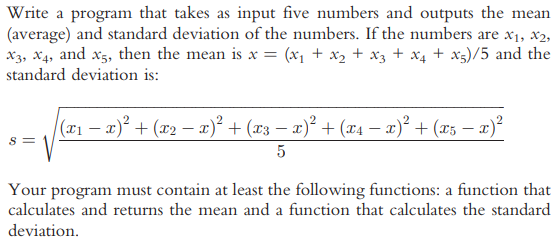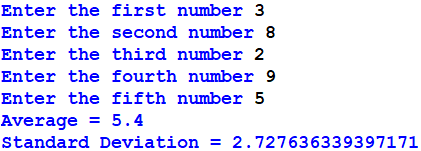## Python Program to calculate mean & standard deviation of user input numbers.Hint: to calculate the square root for a number, import math library and use function sqrt (math.sqrt(number))

Sample output:import math

def average(x1, x2, x3, x4, x5):
x = (x1 + x2 + x3 + x4 + x5)/5

return x

def stdev(x1, x2, x3, x4, x5, x):
s = math.sqrt((((x1-x)**2) + ((x2-x)**2) + ((x3-x)**2) + ((x4-x)**2) + ((x5-x)**2))/5)

return s

x1 = float(input("Enter the first number "))
x2 = float(input("Enter the second number "))
x3 = float(input("Enter the third number "))
x4 = float(input("Enter the fourth number "))
x5 = float(input("Enter the fifth number "))
x = average(x1, x2, x3, x4, x5)
print("Average =", x)
s = stdev(x1, x2, x3, x4, x5, x)
print("Standard Deviation =", s)